# ▼ Part C Calculate the equilibrium concentrations of Na+, Cl ,H+, CHOnd HCHO2 when 50.0 mL of 0.2...▼ Part C Calculate the equilibrium concentrations of Na+, Cl ,H+, CHOnd HCHO2 when 50.0 mL of 0.20 M HCl is mixed with 50.0 mL of 0.20 M NaCHO Express your answers using two significant figures. Enter your answers separated by commas. Request Answer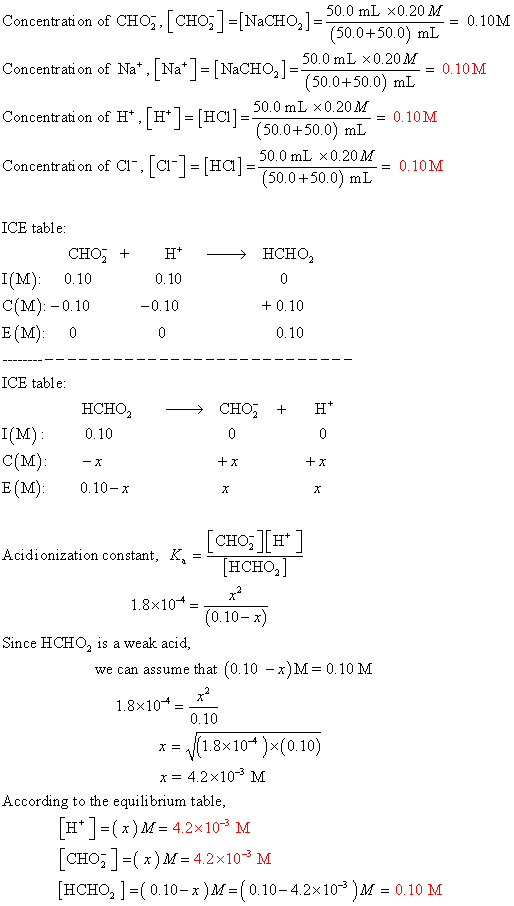##### Add Answer of: ▼ Part C Calculate the equilibrium concentrations of Na+, Cl ,H+, CHOnd HCHO2 when 50.0 mL of 0.2...
Similar Homework Help Questions
• ### Calculate the concentration of all species in a 0.230 M C,H,NH3 Cl solution. Enter your answers...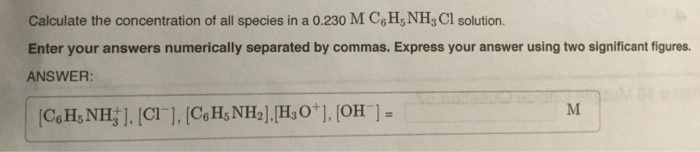Calculate the concentration of all species in a 0.230 M C,H,NH3 Cl solution. Enter your answers numerically separated by commas. Express your answer using two significant figures. ANSWER: М [CeHs NH].[CI ], [CeH,NH2].H,O*]. (O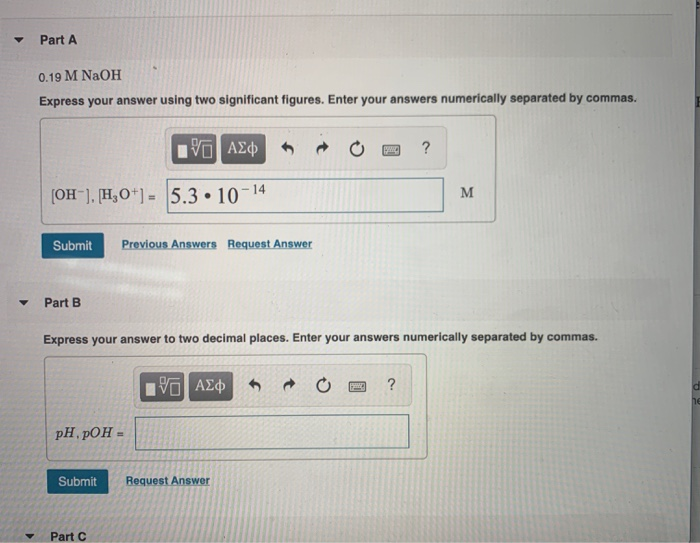Part A 0.19 M NaOH Express your answer using two significant figures. Enter your answers numerically separated by commas. 30 AZ OO ? [OH-], H0+] - 5.3 • 10-14 Submit Previous Answers Request Answer Part B Express your answer to two decimal places. Enter your answers numerically separated by commas. © A ¢ O 2 ? pH. pOH = Submit Request Answer Part 2 Part C 1.4x10-3M Ca(OH), er using two significant figures. Enter your answers numerically shparated by commas....

• ### 180Q2 Calculate the concentrations of all species present (H2CO3, HCO−3, CO2−3, H3O+ and OH−) in 0.019...

180Q2 Calculate the concentrations of all species present (H2CO3, HCO−3, CO2−3, H3O+ and OH−) in 0.019 M H2CO3. pH is 4.04 Express your answers using two significant figures. Enter your answers numerically separated by commas.

• ### Part A If V = 35V  is applied across the whole network (Figure 1) , calculate the...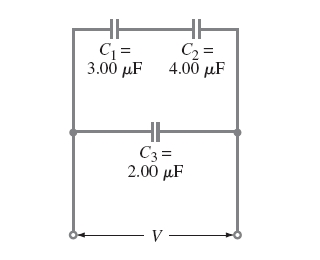Part A If V = 35V  is applied across the whole network (Figure 1) , calculate the voltage across each capacitor. Express your answers using three significant figures separated by commas. V1, V2, V3 = Part B Calculate the charge on each capacitor. Express your answers using three significant figures separated by commas. Q1, Q2, Q3 = If V = 35V is applied across the whole network (Figure 1) , calculate the voltage across each capacitor. Express your answers using three...

• ### Part A: If V = 16V is applied across the whole network(Figure 1) , calculate the...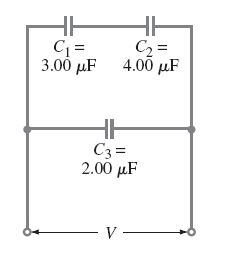Part A: If V = 16V is applied across the whole network(Figure 1) , calculate the voltage across each capacitor. Express your answers using three significant figures separated by commas. (**Answer should be: v1, v2, v3) Part B: Calculate the charge on each capacitor. Express your answers using three significant figures separated by commas. If V = 16V is applied across the whole network(Figure 1) , calculate the voltage across each capacitor. Express your answers using three significant figures separated...

• ### Calculate the equilibrium concentrations: Two sigs figure-Please provide clear steps

At a certain temperature, the reaction has an equilibrium constantPart ACalculate the equilibrium concentrations of ,, and if only is present initially, at a concentration of 0.145 .Express your answers using two significant figures. Enter your answers numerically ceparated by commas., ,=//Could you break it down steps for me to understand how arrive to the conclusion, including clear formulas. Not just summarizing it. Would be helpful if you breakit down in details as I would like to understand it. Thanks....

• ### Assume that 100.0 mL of 0.200 M CsOH and 50.0 mL of 0.400 M HCl are...

Assume that 100.0 mL of 0.200 M CsOH and 50.0 mL of 0.400 M HCl are mixed in a calorimeter. The solutions start out at 22.50 ∘C, and the final temperature after reaction is 24.28 ∘C. The densities of the solutions are all 1.00 g/mL, and the specific heat of the mixture is 4.2J/(g⋅∘C). Calculate the energy which releases in the process. Express your answer using four significant figures.

• ### Calculate: Part A) [H+] for [OH−]=3.5×10^-4 M Express your answer using two significant figures. Part B)...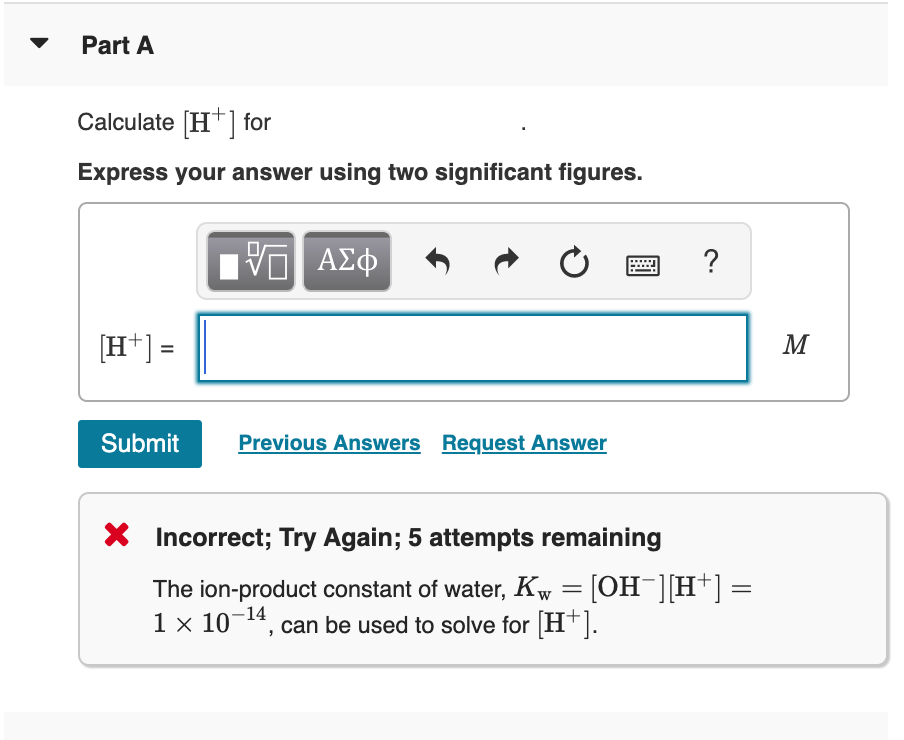Calculate: Part A) [H+] for [OH−]=3.5×10^-4 M Express your answer using two significant figures. Part B) [H+] for [OH−] =8.4×10^-9 M Express your answer using two significant figures. Part C) Calculate [H+] for a solution in which [OH−] is 100 times greater than [H+]. Please show how you solved it, thanks! Part A Calculate (H+] for Express your answer using two significant figures. VO AED ? Submit Previous Answers Request Answer X Incorrect; Try Again; 5 attempts remaining The ion-product...

• ### - Part A ReviewI Constants Periodic Table What are the H,O'] and [OH tor a solution with each of the following pH values? pH 3.2 Express your answers using one significant figure separated by a c...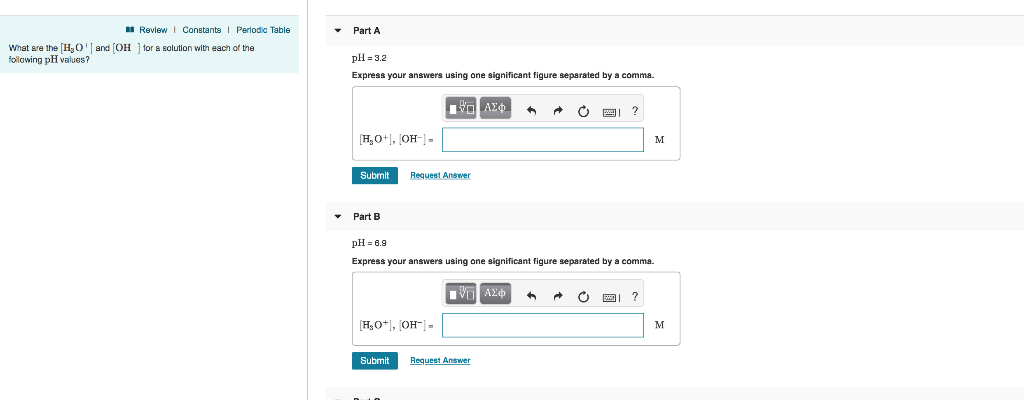- Part A ReviewI Constants Periodic Table What are the H,O'] and [OH tor a solution with each of the following pH values? pH 3.2 Express your answers using one significant figure separated by a comma. Submit Request Answer Part B pH 6.9 Express your answers using one significant figure separated by a comma. Submit Request Answer Review Constants Periodic Table What are the H O ' and [OH following pH values? for a solution with each of the Part...

• ### Indicate the concentration of each ion present in the solution formed by mixing the following. Part...

Indicate the concentration of each ion present in the solution formed by mixing the following. Part A 44.0 mL of 0.110 M Na2SO4 and 25.0 mL of 0.200 M KCl. Assume that the volumes are additive. Enter your answers, separated by commas, using three significant figures. Part B 3.80 g KCl in 75.0 mL of 0.230 M CaCl2 solution. Assume that the volumes are additive. Enter your answers, separated by commas, using three significant figures.

Need Online Homework Help?